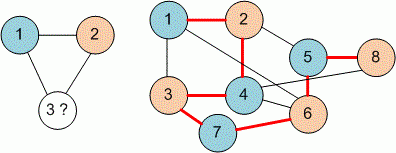Competitions

# Bicoloring

In 1976 the "Four Color Map Theorem" was proven with the assistance of a computer. This theorem states that every map can be colored using only four colors, in such a way that no region is colored using the same color as a neighbor region.

Here you are asked to solve a simpler similar problem. You have to decide whether a given arbitrary connected graph can be bicolored. That is, if one can assign colors (from a palette of two) to the nodes in such a way that no two adjacent nodes have the same color. To simplify the problem you can assume:

• no node will have an edge to itself.
• the graph is nondirected. That is, if a node a is said to be connected to a node b, then you must assume that b is connected to a.
• the graph will be connected. That is, there will be at least one path from any node to any other node.

#### Input

Consists of several test cases. Each test case starts with a line containing the number n (0n1000) of different nodes. The second line contains the number of edges l (1l250000). After this l lines will follow, each containing two numbers that specify an edge between the two nodes that they represent. A node in the graph will be labeled using a number a (1an). The last test contains n = 0 and is not to be processed.

#### Output

You have to decide whether the input graph can be bicolored or not, and print it as shown below.Time limit 2 seconds
Memory limit 128 MiB
Input example #1
3
3
1 2
2 3
3 1
8
12
1 2
2 4
3 4
3 1
3 7
7 6
4 6
1 6
2 5
5 6
4 8
5 8
0

Output example #1
NOT BICOLOURABLE.
BICOLOURABLE.